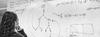# 2nd Semester 2015/16: Probabilistic Logics and Probabilistic Inference

Instructors
ECTS
6
Description
This project aims at investigating probabilistic logics and probabilistic inference. We shall look at the logics that can express and formalise the relation between probabilistic statements as well as logics that use probability functions for the valuation of formulas. Besides from the purely mathematical interest in investigating these logical settings, they have proved to be of great relevance in many fields of application and have become very popular in applications of logic in different disciplines where the study of uncertainty or under-determination becomes relevant.

We will look at the probabilistic logics introduced by Halpern et al, logics with probabilistic modalities, Probabilistic (Dynamic) Epistemic Logic, as well as Inductive Logic and probabilistic inference. The question of interest here is how to assign probabilities (truth values) to arbitrary sentences in the language on the basis of some partial (probabilistic) information. We will in particular look into an axiomatisations of probabilistic inference proposed by Paris and Vencovska and will look into the two most popular inference processes (Maximum Entropy and Centre of Mass) in details. We shall finally develop briefly the connections to formal epistemology and belief revision.

Organisation
The course will be a mixture of introductory lectures, presentations by students and discussion sessions. The main goal of the project is to provide students with an overview of the field and to introduce them to some topics of current interest in this area, both in mathematics and in formal epistemology.

The meetings will take place three times a week (except for the week of June 11when there are no meetings )

Prerequisites
We will assume familiarity with propositional logic and basic knowledge of probability theory. We will not require any advance familiarity with probability theory and most of what is needed will be covered in the lectures. However, such knowledge can be helpful.
Assessment
Students will be evaluated on the basis of presentations and a written report. The report can either be a review of part of the literature or a small original work.